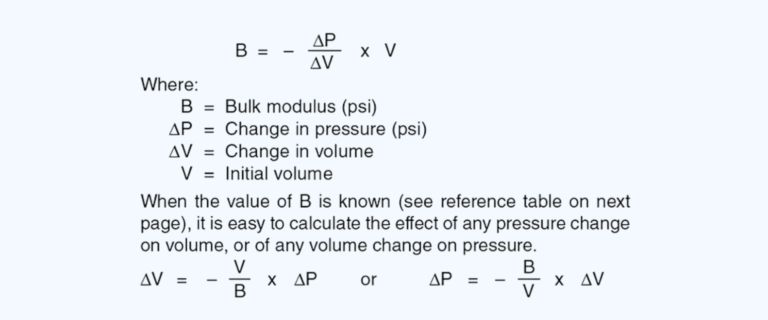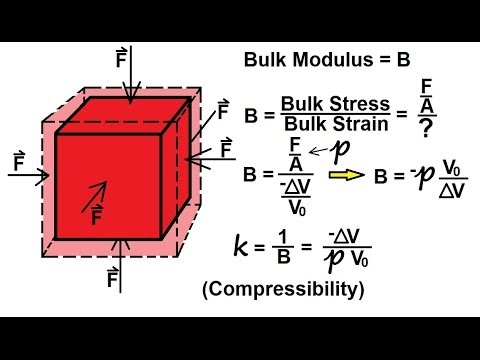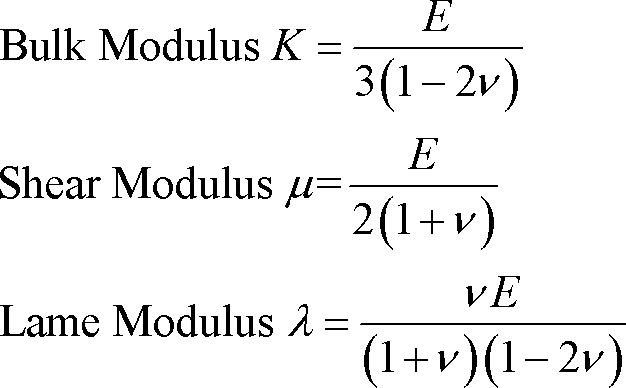# The History of Bulk Modulus: Exploring Material Elasticity

##### The History of Bulk Modulus:

The concept of bulk modulus, a fundamental property of materials, has been of great interest to scientists and engineers for centuries. Bulk modulus measures the resistance of a substance to compression when subjected to external forces. In this article, we will delve into the history of bulk modulus, provide a timeline of its development, answer frequently asked questions, share interesting facts, and highlight the significance of this concept in understanding material elasticity.History of Bulk Modulus:The understanding of bulk modulus can be traced back to the work of renowned physicists and mathematicians over several centuries. The concept emerged as a fundamental aspect of material behavior and elasticity.17th Century: The concept of elasticity was first explored by Robert Hooke, who formulated Hooke's Law in 1660. Hooke's Law describes the linear relationship between the force applied to a material and the resulting deformation.18th Century: The French physicist Edme Mariotte conducted extensive studies on the compressibility of gases. His work laid the foundation for understanding the behavior of materials under compression.19th Century: Augustin-Louis Cauchy, a French mathematician, made significant contributions to the understanding of material elasticity, including the development of equations that relate stress and strain in a material.

 Q: What is bulk modulus? A: Bulk modulus is a measure of a material's resistance to compression. It represents the ratio of the change in pressure applied to a material to the resulting fractional change in volume. Q: How is bulk modulus different from other moduli? A: Bulk modulus is specific to the resistance to compression, whereas Young's modulus measures a material's resistance to stretching and shear modulus measures resistance to shear deformation.
##### Conclusion:

The concept of bulk modulus has evolved over centuries, driven by the quest to understand the behavior of materials under compression. From the foundational work of Hooke, Mariotte, and Cauchy to Lord Kelvin's formal definition of bulk modulus, scientists and engineers have made significant strides in unraveling the complexities of material elasticity. The study of bulk modulus continues to be crucial in various fields, enabling us to design and engineer structures, develop new materials, and comprehend the behavior of matter under compression. As we deepen our understanding of bulk modulus, we gain valuable insights into the fundamental nature of materials and their responses to external forces.

##### Timeline of Bulk Modulus:
 1873: The concept of bulk modulus was formally introduced by Lord Kelvin (William Thomson), a British physicist. Kelvin defined bulk modulus as the ratio of the change in pressure to the fractional change in volume. 20th Century: Further advancements in the understanding of bulk modulus and material elasticity were made through experimental and theoretical research conducted by scientists and engineers.
##### Interesting Facts about Bulk Modulus:
 Bulk modulus values can vary significantly across different materials. For example, gases and liquids generally have low bulk modulus, while solids, especially metals, typically have higher bulk modulus values. The bulk modulus of a material influences its response to external forces and impacts its behavior under compression. Materials with high bulk modulus are stiffer and less compressible. Bulk modulus is utilized in various engineering applications, such as the design of structures, the development of materials with specific properties, and the study of seismic waves and their propagation through the Earth's interior.
##### Image Gallery: